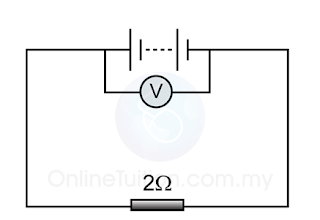# Measuring e.m.f. and Internal Resistance - Open Circuit/Close Circuit

 Open Circuit Close Circuit In open circuit ( when the switch is off), the voltmeter shows the reading of the e.m.f. In close circuit ( when the switch is on), the voltmeter shows the reading of the potential difference across the cell. With the presence of internal resistance, the potential difference across the cell is always less than the e.m.f..

Example 1:

The diagram above shows a simple circuit that connect some baterries to a resistor. The voltmeter shows a reading of 5.0V when the switch is off and 4.5V when the switch is on. What are the e.m.f. and the internal resistance of the cell?

When the switch is off, the reading of the voltmeter shows the e.m.f. of the batteries. Therefore.
e.m.f. = 5.0V

When the switch in turned on, the reading of the voltmeter shows the potential difference of the resistor. Therefore,
V = 4.5V

The current that pass through the resistor,
$I= V R = 4.5 9 =0.5A$
$E=V+Ir (5.0)=(4.5)+(0.5)r 0.5r=5.0−4.5=0.5 r= 0.5 0.5 =1Ω$

Example 2:Diagram (a)Diagram (b)

A cell is connected to a circuit as shown in diagram (a). The graph in diagram (b) shows the change of the reading of the voltmeter, V against time, t. If t is the time where the switch is close, find
(a) the e.m.f. of the cell
(b) the internal resistance of the cell.

$I= V R = 2.5 2 =1.25A$
$E=V+Ir (3.0)=(2.5)+(1.25)r 1.25r=3.0−2.5=0.5 r= 0.5 1.25 =0.4Ω$